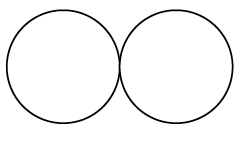### Home > CCG > Chapter 9 > Lesson 9.1.4 > Problem9-47

9-47.While Katarina was practicing her figure skating, she wondered how far she had traveled. She was skating a “figure $8$,” which means she starts between two circles and then travels on the boundary of each circle, completing the shape of a sideways $8$. If both circles have a radius of $5$ feet, how far does she travel when skating one “figure $8$”?

What is the circumference of one circle? How can this help you find the total distance traveled?